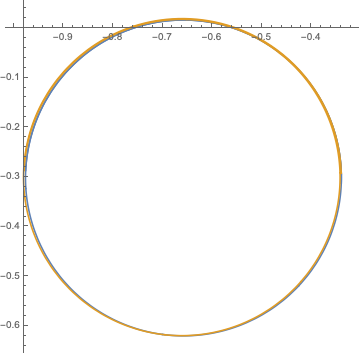# turn $\pi/n$, move $1/n$ forward

start at the origin, first step number is 1.

• turn $$\pi/n$$
• move $$1/n$$ units forwardAngles are cumulative, so this procedure is equivalent (finitely) to $$u(k):=\sum_{n=1}^{k} \frac{\exp(\pi i H_{n})}{n}$$

• Is the limiting shape formed by a line plot of partial sums a circle or a spiral? Relying on visual intuition with the harmonic numbers seems to be perilous.
• Where's its center? This is equivalent to "If $$u(k)$$ converges, what does it converge to?".

Naively resumming within exp produces lots of divergent series as a result of the harmonic numbers $$H_{n}$$. At the cost of being a numerically ill-conditioned sum with lots of cancellation, I have to wonder what this says of arithmetic properties of the harmonic numbers.

The large-$$n$$ asymptotics of the harmonic numbers is $$H_n\simeq \gamma_E+\log n$$, which we use for $$n\geq k_0$$, replacing the sum $$\sum_{n=k_{0}}^k$$ by an integral $$\int_{k_0}^k dn$$. We thus find \begin{align} U(k)&=u(k_0-1)+e^{i\pi\gamma_E}\int_{k_0}^k \frac{1}{n}e^{i\pi\log n}\,dn\\ &=u(k_0-1)+\frac{i}{\pi} e^{i \pi\gamma_E } \left(k_0^{i\pi}-k^{i \pi }\right). \end{align} So $$U(k)$$ traces out a circle in the complex plane, of radius $$1/\pi$$ and center at $$z_0=u(k_0-1)+(i/\pi)e^{i \pi\gamma_E } k_0^{i\pi}$$.
The plot compares $$u(k)$$ (gold) and $$U(k)$$ (blue) for $$k_0=50$$ and $$k$$ up to 1000, when $$z_0=-0.66- 0.28i$$. The agreement is quite satisfactory.• While this suggests that $u(k)$ also converges to a circle of radius $1/\pi$, the centers of the two circles will be unrelated. Aug 14 '19 at 11:05
• The comment by @alpoge may be more easily read as Using $H_n = \log{n} + \gamma + O(1/n)$, $\exp(x + \epsilon) = \exp(x) (1 + O(\epsilon))$, and partial summation (in the form $\int_1^X t^{-1+\pi i} d\lfloor{t}\rfloor = \int_1^X t^{-1+\pi i} dt + O(\int_1^X \{t\} t^{-2+\pi i} dt)$ ), I believe this answer does indeed give an asymptotic (and explains the offset of the centre mentioned above). Aug 14 '19 at 13:07